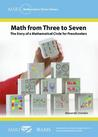# With Educational Games, Even if the Kids Don't Get It, You Have Still Played Together

...where does probability theory come from? What is its source? Clearly, like many other sciences, like arithmetic itself, probability theory emerged from observations of certain real-world phenomena, namely, random, unpredictable phenomena. And it is exactly these kinds of observations—fundamental to the formation of science—which are worth making together with kids. Well, not all of them, of course, just the simplest ones. Besides, kids are making them on their own; e.g., when they play games with dice. What we can do is just make the probabilistic nature of their observations slightly more evident, as well as introduce them to the fact that a probabilistic world is also quite varied. We can show them, instead of a dice, an irregular polyhedron, and they will see that this way the game becomes “unjust”: some scores happen more frequently than others. Or you can propose a game where they have to add the scores on two dice. Here too the kids will sooner or later notice that they get 7 more often than 2. With these kinds of activities the only limits are our imagination and the capacities of kids. If they have understood something, if something has stayed in they head, this is excellent. If not—no problem, then we just “played together.”

## Notes:

Folksonomies: education parenting

Taxonomies:
/science/computer science/artificial intelligence (0.568185)
/travel/tourist facilities/hotel (0.521914)

Keywords:
probability theory (0.972593 (:0.000000)), certain real-world phenomena (0.898535 (:0.000000)), kids (0.762952 (:0.000000)), unpredictable phenomena (0.747349 (:0.000000)), simplest ones (0.745069 (:0.000000)), irregular polyhedron (0.731883 (:0.000000)), Educational Games (0.723355 (:0.000000)), probabilistic nature (0.718410 (:0.000000)), probabilistic world (0.715720 (:0.000000)), dice (0.679794 (:0.000000)), observations (0.558133 (:0.000000)), kinds (0.553175 (:0.000000)), scores (0.524528 (:0.000000)), source (0.474999 (:0.000000)), capacities (0.470833 (:0.000000)), formation (0.468921 (:0.000000)), notice (0.468226 (:0.000000)), sciences (0.465551 (:0.000000)), imagination (0.465405 (:0.000000)), course (0.465153 (:0.000000)), limits (0.463927 (:0.000000)), e.g. (0.462818 (:0.000000)), problem (0.461981 (:0.000000)), fact (0.460755 (:0.000000))

Concepts:
Game (0.980204): dbpedia_resource
Probability (0.907296): dbpedia_resource
Probability theory (0.756632): dbpedia_resource
Event (0.731922): dbpedia_resource
Theory (0.709709): dbpedia_resource
Science (0.699342): dbpedia_resource
Probability space (0.634934): dbpedia_resource
Dice (0.630126): dbpedia_resourceMath from Three to Seven: The Story of a Mathematical Circle for Preschoolers
Books, Brochures, and Chapters>Book:  Zvonkin, Alexander (2006), Math from Three to Seven: The Story of a Mathematical Circle for Preschoolers, Retrieved on 2018-07-27
Folksonomies: education mathematics